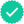#### Related questions

Question

Consider the following.
x = 1 + t2
y = t2 + t3

(a) Find the following.
(dy)/(dx) =

(d^2y)/(dx^2) =

(b) For which values of t is the curve concave upward? (If you need to use or –, enter INFINITY or –INFINITY, respectively.)

Expert SolutionKnowledge Booster••